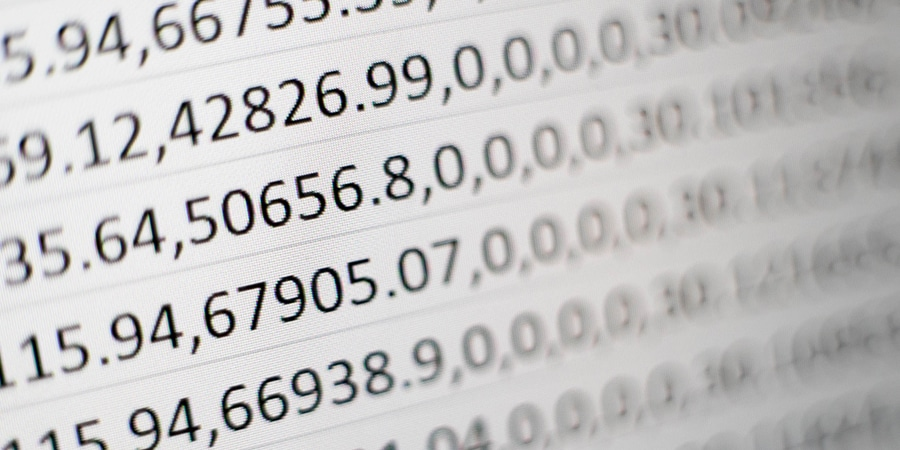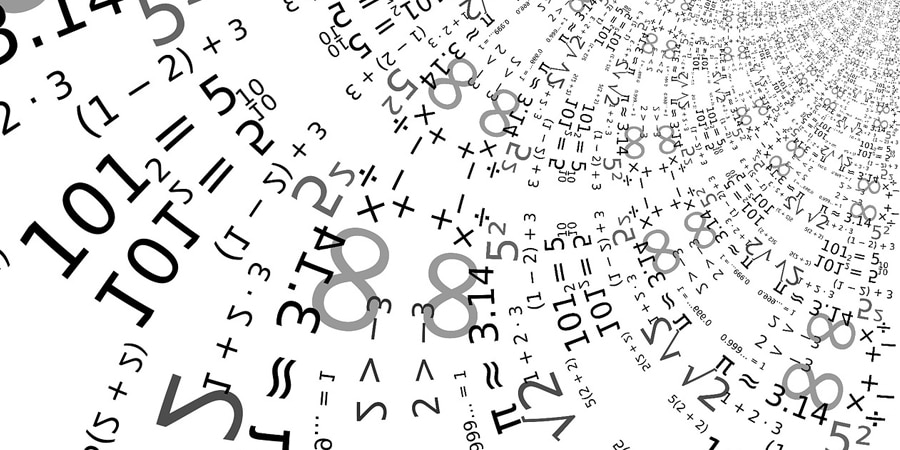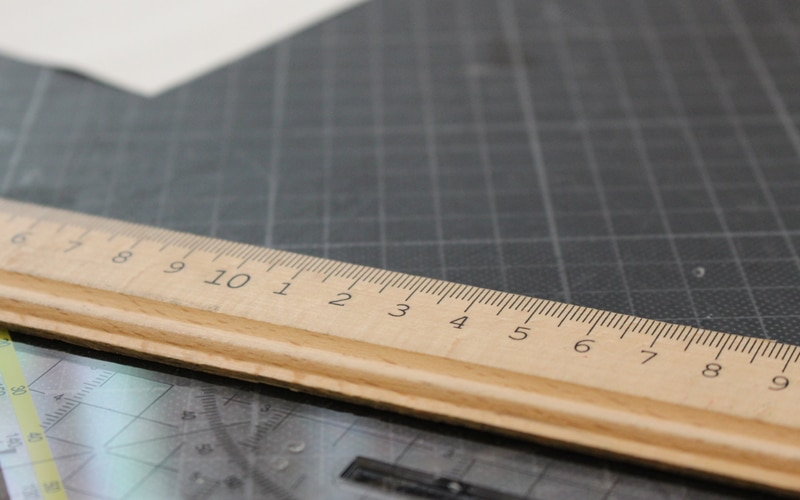# Significant Figures Worksheet(Sig Figs): Definition, Examples, and PracticeMeta: Whether you’re relatively new to significant figures or are just looking for a refresher, you’ve come to the right place. Read on to learn more!

# Significant Figures (Sig Figs): Definition, Examples, and Practice

Significant figures are a vital concept when you’re working in any field that requires precision; chemistry, for example. And while it feels like a complex subject, it’s fairly simple and straightforward. In this article, we’re answering your questions about significant figures and prepping you for significant figures worksheets with lots of examples.

## Reason for Significant FiguresWe can all agree that there’s nothing worse than complicated mathematics concepts that don’t seem to have anything to do with real life. Fortunately, that’s not the case with significant figures! The primary purpose for this somewhat abstract concept is precision.

The numbers we use in everyday life, as it turns, are not that precise. When you’re working in specialized fields, however, the precision of the data is everything. Significant figures help you communicate how precisely you’ve measured and help to ensure mistakes are made with the data.

### Let’s Build a TowerHere’s an example: let’s say you’re building a tower. As you might imagine, precision matters hugely. You don’t want to get to the top of the tower only to realize your tower is taller–or shorter!–than you thought. Let’s say, then, that the steel manufacturer has measured its vertical beams to the nearest centimeter and is sending you three beams that are each 20.5 meters tall.

This measurement is precise to the centimeter, but the number doesn’t tell you anything about millimeters. This is a problem because the glass you’re cutting to cover the building in is measured to the nearest millimeter; in fact, the glass company is sending the glass to you in 15.75-meter sheets.

You know that your steel beams will stand end to end and that your finished tower will be (20.5*3) meters tall, or 61.5 meters tall. Three glass sheets will be 47.25 meters tall; too short. Four glass sheets will be 63 meters tall; too tall. You’ll need to lay three sheets of glass and cut the fourth to size. Now you need to figure out how much you should cut down that fourth sheet of glass.

### The Problem With ImprecisionHere’s the problem: your steel is measured to the centimeter, but the glass cutter cuts to the millimeter. Each steel beam is 20.5 meters, which means it’s twenty meters and five centimeters; but how many millimeters is it?

We don’t know. It could be three millimeters (20.53 meters), or it could be seven millimeters (20.57 meters). Let’s say it’s the latter, and your three steel beams each have an extra seven millimeters that you don’t know about because they’ve only been measured to the nearest centimeter.

You think you’ve only got 61.5 meters of a tower (because 20.5*3 = 61.5) but you have 61.71 meters (because 20.57*3 = 61.71). That’s a difference of .21 meters, or a whopping two centimeters and one millimeter! If you cut your glass to 61.5 meters, you’ll get to the top of the tower to install it–only to realize you’re missing two centimeters and a millimeter of glass.

Oops!

This is an oversimplified example, but it helps to illustrate how important precision is–and now you know how significant figures work, even if you don’t know the concept by that name yet. That’s for the next section!

## Rules for Significant Figures

The first thing you should remember is that significance doesn’t indicate whether a number is computed or not; it simply indicates the precision of measurement. We’ll explain; let’s start with the basic ground rules about significant figures, and then we’ll practice with some examples you might see on a significant figures worksheet:

1. If it’s not a zero, the number is a significant figure (e.g., 1, 2, 3, 4, 5, 6, 7, 8, and 9 are all significant no matter where they are in the number)
2. If a zero is between a non-zero digit, it is significant (e.g., the zeros in these numbers are significant: 507, 5007, 5000.07)
3. Trailing zeros (the zeros at the end of a number) are only significant if the number has a decimal point (e.g., 5.0500 or 5.50); they are not significant if there are no decimal points (e.g., 500 or 50) (special note: in the latter case, significance can be indicated with a bar above or below the last measured figure)
4. Leading zeros (zeros before non-zeros) are never significant (e.g., 005 or 0.005)

### EXAMPLES

• 51 has two significant figures (5 and 1; all numbers are non-zeros)
• 1234.56 has six significant figures (1, 2, 3, 4, 5 and 6; all numbers are non-zeros)
• 505.5505 has seven significant figures (5, 5, 0, 5, 5, 0, and 5; all non-zeros are significant, and all zeros between non-zeros are significant)
• 0.0057 has two significant figures (5 and 7; the zeros are leading and are not significant)
• 500 and 50 each have one significant figure (5 and 5; trailing zeros are not significant unless there is a decimal point)
• 5.0500 has five significant figures (5, 0, 5, 0, and 0; the first zero is between two non-zeros and the trailing zeros are significant because the number contains a decimal)
• 0.00050500 has five significant figures also (remember that leading zeros do not count)
• 1300 has two significant figures (one and three) while 1300. has four significant figures (1, 3, 0, and 0) because the latter number has a decimal point

## Multiplying, Dividing, Adding, and Subtracting with Significant Figures

Before we get into the mechanics of multiplying, dividing, adding, and subtracting with significant figures, you’ll first need to know how to round up significant figures. For example, if you have a number (let’s say 54.896) that has five significant figures, and you need to round it up to three significant figures, how do you do that?

### Rounding Up

Here’s how:

1. Identify the significant figures (in our example, there are five significant numbers: 5, 4, 8, 9, and 6)
2. Identify the last of the desired significant figures (we know we need three significant figures, so eight, the third significant figure from the left, is the last significant figure)
3. Look at the number to the right of the significant figure. If it is five or greater, add one to the last significant figure (in our example, eight is followed by nine; nine is greater than 5; therefore, eight is rounded up by 1 to 9 so that our new number is 54.9). If, however, the number is four or less, simply remove all the remaining numbers to the left (don’t replace them with zeros)

Here’s another example using 0.01234500:

If you want to round it to three significant figures, the answer would be 0.0123 (leading zeros are not significant; 4 is less than five, so 4 and all subsequent numbers are removed).

Special note: rounding significant figures is different than rounding decimals. For example, if you wanted to round our example number to three decimal places, the answer would be .012, which is a different number altogether from a precision standpoint.

### Multiplying and Dividing

When you begin working seriously with multiplying and dividing, you’ll notice how quickly things can move from precise to imprecise. For example, let’s go back to our tower example and say we want to measure the area of our glass sheets. They are 15.75 meters tall and 2.5 meters wide.

A simple calculation (15.75*2.5=39.375) tells us the area is 39.375, but if you’re thinking, “wait! That measurement is more precise than what we measured!” you’d be right. To keep our quotients and products as precise as our initial measurements, we need to round up (or down).

The general rule is that the significant figures in the answer cannot be more than the smallest amount of significant figures used in the equation. In our glass sheets example, the two numbers we multiplied are 15.75 (which has four significant figures) and 2.5 (which has two significant figures). The smallest number of significant figures in either of these factors is two, so our answer can’t have more than two significant figures.

Our answer (39.375) has five significant figures, with 9 being the second significant figure. The number to the right of 9 is 3, which is less than 5, so we simply discard 3 and everything to its right. Our answer, then, is 40. (9 rolls to the 0 and the 3 becomes a 4; also note the use of the decimal!).